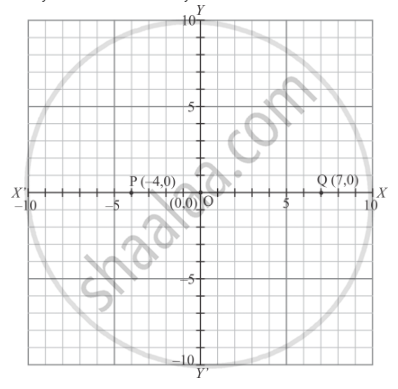# Points (−4, 0) and (7, 0) Lie - Mathematics

MCQ

Points (−4, 0) and (7, 0) lie

• on x-axis

• y-axis

#### Solution

Let the points P and Q whose coordinates are  (−4, 0) and  (7, 0) respectively. Locate the points and you will see that they lie on x-axis.Concept: Coordinate Geometry
Is there an error in this question or solution?
Chapter 8: Co-ordinate Geometry - Exercise 8.2 [Page 7]

#### APPEARS IN

RD Sharma Mathematics for Class 9
Chapter 8 Co-ordinate Geometry
Exercise 8.2 | Q 5 | Page 7

Share